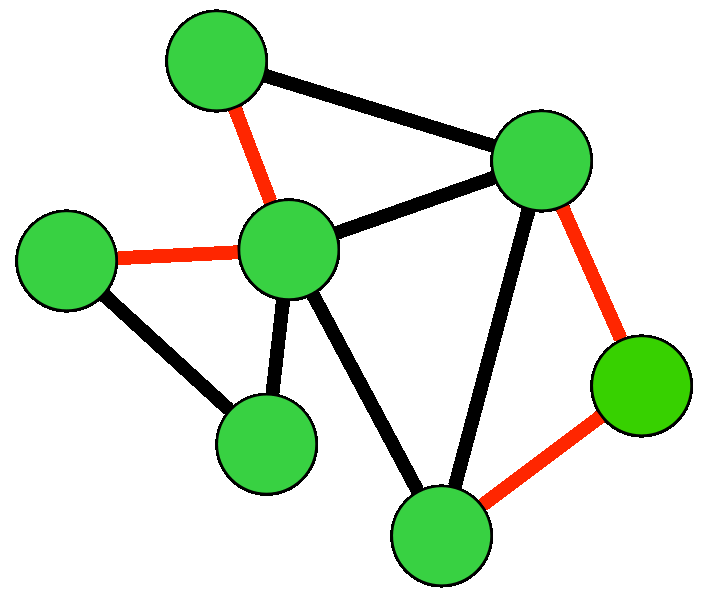## Consensus with Link Failures/Packet Losses

Consensus with random link failures is a prototype problem for studying the dynamics of stochastic networks. In its simplest form, this problem can be recast as linear systems with multiplicative noise, or equivalently as uncertain systems with structured stochastic uncertainty. Such systems can exhibit complex phenomena such as heavy tailed distributions and Levy flights, yet are more tractable than completely general stochastic systems.

### The network setting

Consider a consensus algorithm over a network in which links randomly fail at each round. These link failures can represent either communication failures between nodes andor packet losses. We thus have a consensus algorithm over a type of stochastic network/. This setting is caricatured in the following animated diagram (red color depicts failing links).We are interested in not just whether consensus is asymptotically achieved or not, but rather more detailed statements about the rate of convergence as a function of probability of failure, and network topology parameters. In our stochastic setting, this is quantified by the variance of deviation from average.

### The system theory reformulation

A nice feature of this problem is that the dynamics of the standard average consensus algorithm over a network with link failures can be recast as a linear system with multiplicative noise. The dynamics look like thiswhere the deltas are iid bernouli random variables representing the failures of each of the n links. The B matrices represent the effect of each link's disconnection on the algorithm's dynamics.

This is now a stochastic system, but a recursion equation for the covariance of the state can be derived to bewhere the sigmas are the variances of each of the deltas. The actual recursion we use is a little different since one needs to keep track of the covariances of the deviation from average state, rather than the actual state, but the basic idea is similar. All second order convergence properties of the stochastic system (such as rates of convergece of variances) can be deduced from studying this matrix recursion equation.

### The mathematical tools used

Convergence properties of the covariance recursion are determined by studying the Lyapunov-like matrix-valued operatorFor example, the eigenvalues of this operator determine the convergence rate of variances. In general, there is no simple relationship between the eigenvalues of this operator and the spectral properties of the originalmatrices (e.g. one cannot easily relate the second smallest graph Laplacian eigenvalue, or the Fiedler vector, to the eigenvalues of this operator).

Our approach is to use spectral perturbation theory and derive the convergence rates up to first order in the probability of failure. For certain regular graph structures, we can obtain explicit expressions for these rates.

## Reformulation as a stochastic structured uncertainty problem

Problems with multiplicative uncertainty can be viewed in a unified manner as structured uncertainty problems as shown in the diagram below.This is the point of view that originated in the robust control literature in the early 80s. The case of stochastic deltas is a little more recent in the work of Lu & Skelton and Elia. The necessary and sufficient condition is a bound on the spectral radius of the matrix of subsystems’norms. We can recast the link failure problem in this setting. A particularly nice feature is that the network's structure and topology translates to a special structure in the nominal system M. Thus, more refined statements about the spectral radius of the matrix of norms can be made. See the last two papers below for the details.

## Related Papers

• Bassam Bamieh, Structured Stochastic Uncertainty, Proceedings of the 50th Annual Allerton Conference on Communications, Control, and Computing, pp. 1498-1503, 2012.